# 8.3 Multiple bonds  (Page 2/2)

 Page 2 / 2

In molecules with sp hybrid orbitals, two unhybridized p orbitals remain on the atom ( [link] ). We find this situation in acetylene, $\text{H−C≡C−H},$ which is a linear molecule. The sp hybrid orbitals of the two carbon atoms overlap end to end to form a σ bond between the carbon atoms ( [link] ). The remaining sp orbitals form σ bonds with hydrogen atoms. The two unhybridized p orbitals per carbon are positioned such that they overlap side by side and, hence, form two π bonds. The two carbon atoms of acetylene are thus bound together by one σ bond and two π bonds, giving a triple bond.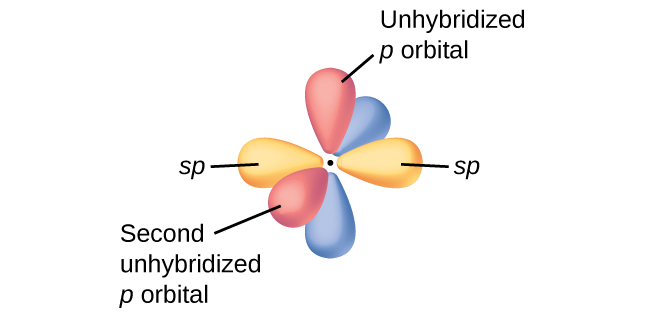Diagram of the two linear sp hybrid orbitals of a carbon atom, which lie in a straight line, and the two unhybridized p orbitals at perpendicular angles.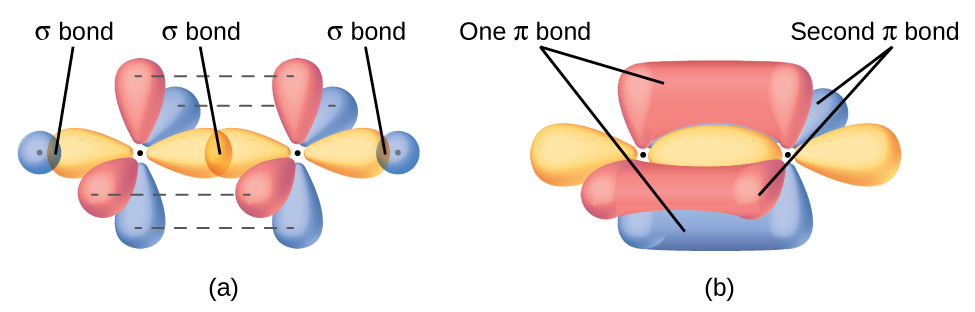(a) In the acetylene molecule, C 2 H 2, there are two C–H σ bonds and a C ≡ C triple bond involving one C–C σ bond and two C–C π bonds. The dashed lines, each connecting two lobes, indicate the side-by-side overlap of the four unhybridized p orbitals. (b) This shows the overall outline of the bonds in C 2 H 2 . The two lobes of each of the π bonds are positioned across from each other around the line of the C–C σ bond.

Hybridization involves only σ bonds, lone pairs of electrons, and single unpaired electrons (radicals). Structures that account for these features describe the correct hybridization of the atoms. However, many structures also include resonance forms. Remember that resonance forms occur when various arrangements of π bonds are possible. Since the arrangement of π bonds involves only the unhybridized orbitals, resonance does not influence the assignment of hybridization.

For example, molecule benzene has two resonance forms ( [link] ). We can use either of these forms to determine that each of the carbon atoms is bonded to three other atoms with no lone pairs, so the correct hybridization is sp 2 . The electrons in the unhybridized p orbitals form π bonds. Neither resonance structure completely describes the electrons in the π bonds. They are not located in one position or the other, but in reality are delocalized throughout the ring. Valence bond theory does not easily address delocalization. Bonding in molecules with resonance forms is better described by molecular orbital theory. (See the next module.)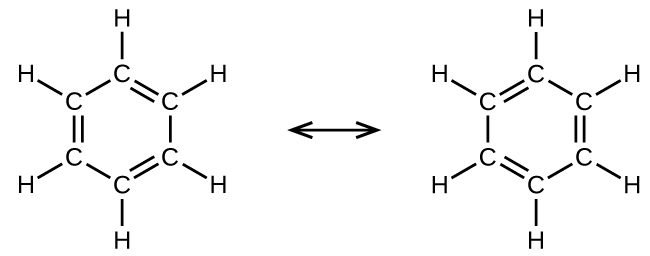Each carbon atom in benzene, C 6 H 6 , is sp 2 hybridized, independently of which resonance form is considered. The electrons in the π bonds are not located in one set of p orbitals or the other, but rather delocalized throughout the molecule.

## Assignment of hybridization involving resonance

Some acid rain results from the reaction of sulfur dioxide with atmospheric water vapor, followed by the formation of sulfuric acid. Sulfur dioxide, SO 2 , is a major component of volcanic gases as well as a product of the combustion of sulfur-containing coal. What is the hybridization of the S atom in SO 2 ?

## Solution

The resonance structures of SO 2 areThe sulfur atom is surrounded by two bonds and one lone pair of electrons in either resonance structure. Therefore, the electron-pair geometry is trigonal planar, and the hybridization of the sulfur atom is sp 2 .

## Check your learning

Another acid in acid rain is nitric acid, HNO 3 , which is produced by the reaction of nitrogen dioxide, NO 2 , with atmospheric water vapor. What is the hybridization of the nitrogen atom in NO 2 ? (Note: the lone electron on nitrogen occupies a hybridized orbital just as a lone pair would.)

sp 2

## Key concepts and summary

Multiple bonds consist of a σ bond located along the axis between two atoms and one or two π bonds. The σ bonds are usually formed by the overlap of hybridized atomic orbitals, while the π bonds are formed by the side-by-side overlap of unhybridized orbitals. Resonance occurs when there are multiple unhybridized orbitals with the appropriate alignment to overlap, so the placement of π bonds can vary.

## Chemistry end of chapter exercises

The bond energy of a C–C single bond averages 347 kJ mol −1 ; that of a $\text{C}\equiv \text{C}$ triple bond averages 839 kJ mol −1 . Explain why the triple bond is not three times as strong as a single bond.

A triple bond consists of one σ bond and two π bonds. A σ bond is stronger than a π bond due to greater overlap.

For the carbonate ion, ${\text{CO}}_{3}{}^{2-},$ draw all of the resonance structures. Identify which orbitals overlap to create each bond.

A useful solvent that will dissolve salts as well as organic compounds is the compound acetonitrile, H 3 CCN. It is present in paint strippers.

(a) Write the Lewis structure for acetonitrile, and indicate the direction of the dipole moment in the molecule.

(b) Identify the hybrid orbitals used by the carbon atoms in the molecule to form σ bonds.

(c) Describe the atomic orbitals that form the π bonds in the molecule. Note that it is not necessary to hybridize the nitrogen atom.

(a)(b) The terminal carbon atom uses sp 3 hybrid orbitals, while the central carbon atom is sp hybridized. (c) Each of the two π bonds is formed by overlap of a 2 p orbital on carbon and a nitrogen 2 p orbital.

For the molecule allene, ${\text{H}}_{2}\text{C}=\text{C}={\text{CH}}_{2},$ give the hybridization of each carbon atom. Will the hydrogen atoms be in the same plane or perpendicular planes?

Identify the hybridization of the central atom in each of the following molecules and ions that contain multiple bonds:

(a) ClNO (N is the central atom)

(b) CS 2

(c) Cl 2 CO (C is the central atom)

(d) Cl 2 SO (S is the central atom)

(e) SO 2 F 2 (S is the central atom)

(f) XeO 2 F 2 (Xe is the central atom)

(g) ${\text{ClOF}}_{2}{}^{\text{+}}$ (Cl is the central atom)

(a) sp 2 ; (b) sp ; (c) sp 2 ; (d) sp 3 ; (e) sp 3 ; (f) sp 3 d ; (g) sp 3

Describe the molecular geometry and hybridization of the N, P, or S atoms in each of the following compounds.

(a) H 3 PO 4 , phosphoric acid, used in cola soft drinks

(b) NH 4 NO 3 , ammonium nitrate, a fertilizer and explosive

(c) S 2 Cl 2 , disulfur dichloride, used in vulcanizing rubber

(d) K 4 [O 3 POPO 3 ], potassium pyrophosphate, an ingredient in some toothpastes

For each of the following molecules, indicate the hybridization requested and whether or not the electrons will be delocalized:

(a) ozone (O 3 ) central O hybridization

(b) carbon dioxide (CO 2 ) central C hybridization

(c) nitrogen dioxide (NO 2 ) central N hybridization

(d) phosphate ion $\left({\text{PO}}_{4}{}^{3-}\right)$ central P hybridization

(a) sp 2 , delocalized; (b) sp , localized; (c) sp 2 , delocalized; (d) sp 3 , delocalized

For each of the following structures, determine the hybridization requested and whether the electrons will be delocalized:

(a) Hybridization of each carbon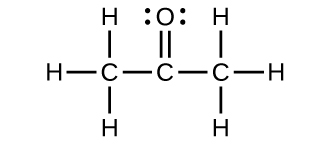(b) Hybridization of sulfur(c) All atoms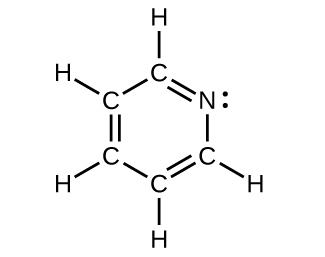Draw the orbital diagram for carbon in CO 2 showing how many carbon atom electrons are in each orbital.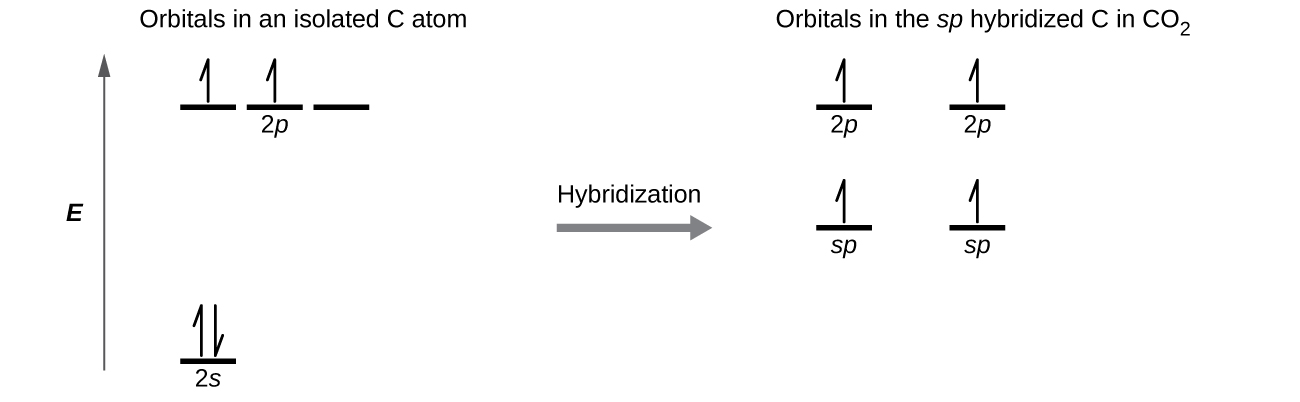Each of the four electrons is in a separate orbital and overlaps with an electron on an oxygen atom.

#### Questions & Answers

to what volume must 8.32 NaOH be diluted to its analytical concentration 0.20 M
weight in mg 1.76 mole of I
Sheriza
the types of hydrocarbons
u are mad go and open textbook
Emmanuel
hahahahahahahahahahahahaha
Jessica
aliphatic and aromatic hydrocarbons
Osakue
I don't use to see the messages
how can you determine the electronegativity of a compound or in molecules
when u move from left to right in a periodic table the negativity increases
reeza
Are you trying to say that the elctronegativity increases down the group and decreases across the period?
Ohanaka
yes and also increases across the period
reeza
for instance when you look at one group of elements in a periodic table electronegativity decreases when you go across the table electronegativity increases. hydrogen is more electronegative than sodium, potassium of that group. oxygen is more electronegative than carbon.
reeza
i hope we all know that organic compounds have carbon as their back bone
OK,Thank you so much for the answer. I am happy now
can I ask you a question now
Osakue
yes
hanna
what is the oxidation number of nitrogen, oxygen and sulphur
Osakue
5, -2 & -2
hanna
What is an atom?
is a smallest particle of a chemical element that can exist
Osakue
can I ask a question
Osakue
it is a substance that cannot be broken down into simpler units by any chemical reaction
An atom is the smallest part of an element dat can take part in chemical reaction.
Idris
an atom is the smallest part of an element that can take part in a chemical reaction nd still retain it chemical properties
Precious
Is the smallest particles of an element that take part in chemical reaction without been change
John
what are the branches of an atomic mass
Still waiting for answers for a very long time now
that question is very strong oooo
Osakue
most of the questions I asked wasn't answered what's the problem guys?
hi, there is no problems ooo
Osakue
between H2SO4 and HCL which is the strongest dehydrating agent ?
Ibirogba
HCl is the strongest dehydrating agent
Osakue
ᴡʜᴀᴛ ᴡɪʟʟ ᴏʙsᴇʀᴠᴇᴅ ɪғ ʟᴇᴀᴅ(ɪɪ)ɴɪᴛʀᴀᴛᴇs ɪs ᴀᴅᴅᴇᴅ ᴏɴ ᴛᴏ sᴏᴅɪᴜᴍ ɪᴏᴅɪᴅᴇ sᴏʟᴜᴛɪᴏɴ
what is the functional group of alkanals
can someone explain salt analysis properly
Frankyx
Find the number of calcium atoms present in a sample weighing 2.0*10 raise to the power of -3g
What does avogadro's law states?
what is chemistry?
Is a branch of science that deals with matter in relation to energy
Kata
permanent hardness of water can be removed by ?
***watertreatmentbasics.com/temporary-and-permanent-hardness-of-water/
caco3
Owoyomi
ʜᴏᴡ
Gawaar
What are the basic area in in chemistry
acid, base and salt
John
Why do people study chemistry
John
becuz chemistry is the study of elements, nature and etc
John
and also the study of matter and atom
John
is chemistry hard
the answer to that question depends on you
John
what kind of question are u asking fam?
Ibirogba
his asking if chemistry is hard
John
not really just a matter of you willing to study
OkorieByByBy